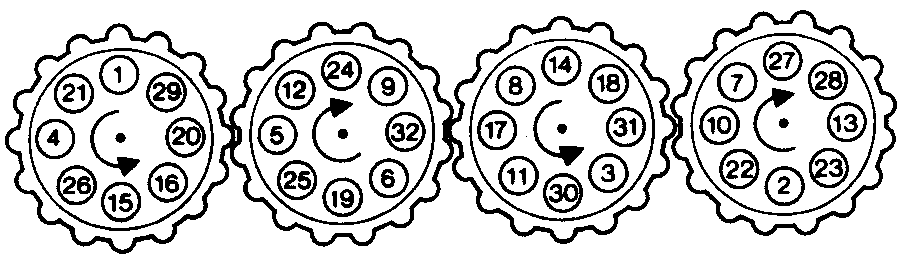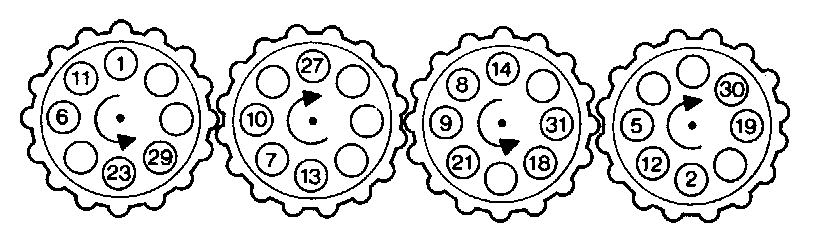#### You may also like### Counting Factors

Is there an efficient way to work out how many factors a large number has?### Summing Consecutive Numbers

15 = 7 + 8 and 10 = 1 + 2 + 3 + 4. Can you say which numbers can be expressed as the sum of two or more consecutive integers?### Helen's Conjecture

Helen made the conjecture that "every multiple of six has more factors than the two numbers either side of it". Is this conjecture true?

# The Patent Solution

##### Age 11 to 14 Challenge Level:

This is quite a challenge and it is really a magic square in disguise! Here is one solution and another similar puzzle for you to try. You may like to study the articles Magic Squares and Magic Squares II.

#### Patent Solution solution#### Another Patent Puzzle - again the magic total is 132

The following puzzle comes from The Ultimate Book of Number Puzzles by Kenneth Kelsey published by The Cresset Press 1992, ISBN: 0 09 177204 4.